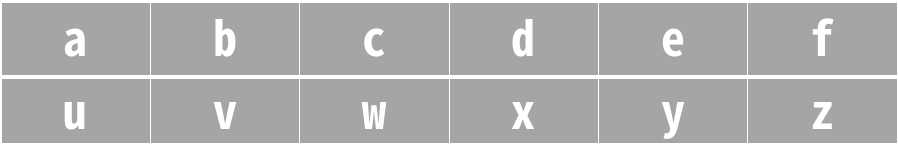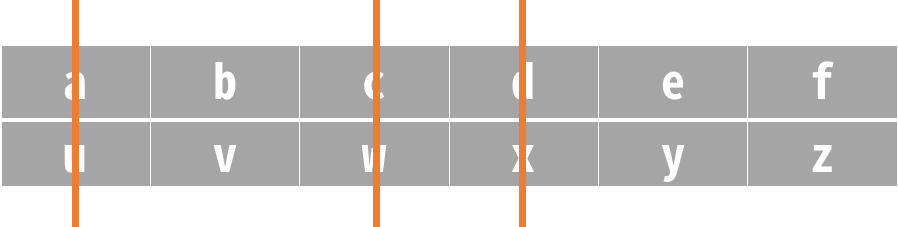LeetCode: 944. 删列造序¶

1、题目描述¶• $1 <= A.length <= 100$

• $1 <= A[i].length <= 1000$

2、解题思路¶

class Solution:
def minDeletionSize(self, A: [str]) -> int:
count = 0
for i in range(len(A)):
temp = []
for j in range(len(A)):
temp.append(A[j][i])

if sorted(temp) != temp:
count += 1
return count